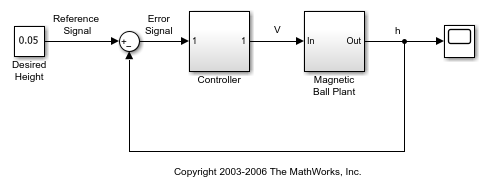# operpoint

Create operating point for Simulink model

## Syntax

```op = operpoint(sys) ```

## Description

`op = operpoint(sys) ` returns an object, `op`, containing the operating point of a Simulink® model, `sys`. Specify `sys` as either a character vector or a string. Use the object with the function `linearize` to create linearized models. The operating point object has the following properties:

• `Model` — Simulink model name, specified as a character vector.

• `States` — State operating point specification, specified as a structure array. Each structure in the array represents the supported states of one Simulink block. (For a list of supported states for operating point objects, see Simulink Model States Included in Operating Point Object.) Edit the properties of this object using dot notation or the `set` function.

Each `States` structure has the following fields:

 `Nx`(read only) Number of states in the Simulink block. `Block` Simulink block name. `StateName` Name of state, specified as a character vector. `x` Simulink block state values, specified as a vector of states. This vector includes all supported states. When creating state value specifications for operating point searches using `findop` and you set the value of a state that you want fixed, also set the `Known` field of the `States` property for that state to `1`. `Ts` (Only for discrete-time states) Sample time and offset of each Simulink block state, specified as a vector. `SampleType` State time rate, specified as one of the following values:`'CSTATE'` — Continuous-time state`'DSTATE'` — Discrete—time state `inReferencedModel` Vector indicating whether each state is inside a reference model:`1` — State is inside a reference model`0` — State is in the current model file `Description` Block state description, specified as a character vector.
• `Inputs` — Input level at the operating point, specified as a vector of input objects. Each input object represents the input levels of one root-level inport block in the Simulink block.

Each entry in `Inputs` has the following fields:

 `Block` Inport block name. `PortWidth` Number of inport block signals. `PortDimensions` Dimension of signals accepted by the inport. `u` Inport block input levels at the operating point, specified as a vector of input levels.When creating input specifications for operating-point searches using `findop`, also set the `Known` field of the `Inputs` property for known input levels that remain fixed during operating point search. `Description` Inport block input description, specified as a character vector.
• `Time` — Times at which any time-varying functions in the model are evaluated, specified as a vector.

• `Version` — Object version number.

## Examples

collapse all

```open_system('magball') ```Create operating point for the model.

```op = operpoint('magball') ```
``` Operating point for the Model magball. (Time-Varying Components Evaluated at time t=0) States: ---------- (1.) magball/Controller/PID Controller/Filter/Cont. Filter/Filter x: 0 (2.) magball/Controller/PID Controller/Integrator/Continuous/Integrator x: 14 (3.) magball/Magnetic Ball Plant/Current x: 7 (4.) magball/Magnetic Ball Plant/dhdt x: 0 (5.) magball/Magnetic Ball Plant/height x: 0.05 Inputs: None ---------- ```

`op` lists each block in the model that has states. There are no root-level inports in this model, therefore `op` does not contain inputs.

You can create new `operpoint` variables in three ways:

• Using the `operpoint` command

• Using assignment with the equals (`=`) operator

• Using the `copy` command

Using the `=` operator results in linked variables that both point to the same underlying data. Using the `copy` command results in an independent `operpoint` object. In this example, create `operpoint` objects both ways, and examine their behavior.

```mdl = 'watertank'; open_system(mdl); op1 = operpoint(mdl)```
``` Operating point for the Model watertank. (Time-Varying Components Evaluated at time t=0) States: ---------- (1.) watertank/PID Controller/Integrator/Continuous/Integrator x: 0 (2.) watertank/Water-Tank System/H x: 1 Inputs: None ---------- ```

Create a new operating-point object using assignment with the `=` operator.

`op2 = op1;`

`op2` is an `operpoint` object that points to the same underlying data as `op1`. Because of this link, you cannot independently change properties of the two `operpoint` objects. To see this, change a property of `op2`. For instance, change the value for the first state from 0 to 2. The change shows in the `States` section of the display.

`op2.States(1).x = 2`
``` Operating point for the Model watertank. (Time-Varying Components Evaluated at time t=0) States: ---------- (1.) watertank/PID Controller/Integrator/Continuous/Integrator x: 2 (2.) watertank/Water-Tank System/H x: 1 Inputs: None ---------- ```

Examine the display of `op1` to see that the corresponding property value of `op1` also changes from 0 to 2.

`op1`
``` Operating point for the Model watertank. (Time-Varying Components Evaluated at time t=0) States: ---------- (1.) watertank/PID Controller/Integrator/Continuous/Integrator x: 2 (2.) watertank/Water-Tank System/H x: 1 Inputs: None ---------- ```

To create an independent copy of an operating-point object, use the `copy` command.

`op3 = copy(op1);`

Now, when you change a property of `op3`, `op1` does not change. For instance, change the value for the first state from 2 to 4.

`op3.States(1).x = 4`
``` Operating point for the Model watertank. (Time-Varying Components Evaluated at time t=0) States: ---------- (1.) watertank/PID Controller/Integrator/Continuous/Integrator x: 4 (2.) watertank/Water-Tank System/H x: 1 Inputs: None ---------- ```

In `op1`, the corresponding value remains 2.

`op1.States(1).x`
```ans = 2 ```

This copy behavior occurs because `operpoint` is a handle object. For more information about handle objects, see Handle Object Behavior (MATLAB).

## Tips

• You can create new `operpoint` variables of in 3 ways:

• Construct a new object with the `operpoint` command.

• Create a new variable by assignment with the equals (=) operator.

• Copy an `operpoint` object using the `copy` command.

Using `operpoint` or `copy` creates a new, independent object. When you use assignment, there is a link between the old and new variable. For an example, see Copy an Operating Point.

## Alternatives

As an alternative to the `operpoint` function, compute operating points to meet your application specifications. For more information, see Compute Steady-State Operating Points.